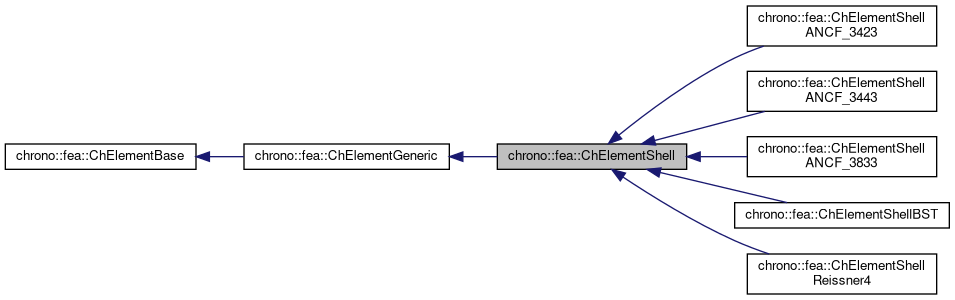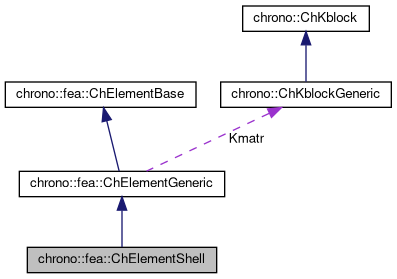chrono::fea::ChElementShell Class Referenceabstract

## Description

Base class for most structural elements of 'shell' type.

#include <ChElementShell.h>

Inheritance diagram for chrono::fea::ChElementShell:[legend]
Collaboration diagram for chrono::fea::ChElementShell:[legend]

## Public Member Functions

virtual void EvaluateSectionDisplacement (const double u, const double v, ChVector<> &u_displ, ChVector<> &u_rotaz)=0
Gets the xyz displacement of a point on the shell, and the rotation RxRyRz of section reference, at parametric coordinates 'u' and 'v'. More...

virtual void EvaluateSectionFrame (const double u, const double v, ChVector<> &point, ChQuaternion<> &rot)=0
Gets the absolute xyz position of a point on the shell, and the absolute rotation of section reference, at parametric coordinates 'u' and 'v'. More...

virtual void EvaluateSectionPoint (const double u, const double v, ChVector<> &point)=0
Gets the absolute xyz position of a point on the shell, at parametric coordinates 'u' and 'v'. More...

virtual void EvaluateSectionVelNorm (double U, double V, ChVector<> &Result)=0
Virtual method to plot velocity field distribution. More...

virtual bool IsTriangleShell ()
Return false if quadrilateral shell - hence u,v parametric coordinates assumed in -1..+1, return true if triangular shell - hence u,v are triangle natural coordinates assumed in 0..+1.Public Member Functions inherited from chrono::fea::ChElementGeneric
ChKblockGenericKstiffness ()
Access the proxy to stiffness, for sparse solver.

virtual void EleIntLoadResidual_F (ChVectorDynamic<> &R, const double c) override
(This is a default (a bit unoptimal) book keeping so that in children classes you can avoid implementing this EleIntLoadResidual_F function, unless you need faster code)

virtual void EleIntLoadResidual_Mv (ChVectorDynamic<> &R, const ChVectorDynamic<> &w, const double c) override
(This is a default (VERY UNOPTIMAL) book keeping so that in children classes you can avoid implementing this EleIntLoadResidual_Mv function, unless you need faster code.)

virtual void EleIntLoadResidual_F_gravity (ChVectorDynamic<> &R, const ChVector<> &G_acc, const double c) override
(This is a default (VERY UNOPTIMAL) book keeping so that in children classes you can avoid implementing this EleIntLoadResidual_F_gravity function, unless you need faster code. More...

virtual void ComputeGravityForces (ChVectorDynamic<> &Fg, const ChVector<> &G_acc) override
(This is the default implementation (POTENTIALLY INEFFICIENT) so that in children classes you can avoid implementing this ComputeGravityForces() function, unless you need faster code.) This fallback implementation uses a temp ChLoaderGravity that applies the load to elements only if they are inherited by ChLoadableUVW so it can use GetDensity() and Gauss quadrature.

virtual void ComputeMmatrixGlobal (ChMatrixRef M) override
Returns the global mass matrix. More...

virtual void InjectKRMmatrices (ChSystemDescriptor &mdescriptor) override
Tell to a system descriptor that there are item(s) of type ChKblock in this object (for further passing it to a solver)

virtual void KRMmatricesLoad (double Kfactor, double Rfactor, double Mfactor) override
Adds the current stiffness K and damping R and mass M matrices in encapsulated ChKblock item(s), if any. More...

virtual void VariablesFbLoadInternalForces (double factor=1.) override
Adds the internal forces, expressed as nodal forces, into the encapsulated ChVariables, in the 'fb' part: qf+=forces*factor (This is a default (a bit unoptimal) book keeping so that in children classes you can avoid implementing this VariablesFbLoadInternalForces function, unless you need faster code)

virtual void VariablesFbIncrementMq () override
Adds M*q (internal masses multiplied current 'qb') to Fb, ex. More...Public Member Functions inherited from chrono::fea::ChElementBase
virtual int GetNnodes ()=0
Gets the number of nodes used by this element.

virtual int GetNdofs ()=0
Gets the number of coordinates in the field used by the referenced nodes. More...

virtual int GetNodeNdofs (int n)=0
Get the number of coordinates from the n-th node that are used by this element. More...

virtual std::shared_ptr< ChNodeFEAbaseGetNodeN (int n)=0
Access the nth node.

virtual void GetStateBlock (ChVectorDynamic<> &mD)=0
Fills the D vector with the current field values at the nodes of the element, with proper ordering. More...

virtual void ComputeNodalMass ()
Compute element's nodal masses.

virtual void ComputeKRMmatricesGlobal (ChMatrixRef H, double Kfactor, double Rfactor=0, double Mfactor=0)=0
Sets H as the stiffness matrix K, scaled by Kfactor. More...

virtual void ComputeInternalForces (ChVectorDynamic<> &Fi)=0
Computes the internal forces (ex. More...

virtual void Update ()
Update: this is called at least at each time step. More...

virtual void EleDoIntegration ()
This is optionally implemented if there is some internal state that requires integration.

## Protected Attributes

double massProtected Attributes inherited from chrono::fea::ChElementGeneric
ChKblockGeneric Kmatr

## ◆ EvaluateSectionDisplacement()

 virtual void chrono::fea::ChElementShell::EvaluateSectionDisplacement ( const double u, const double v, ChVector<> & u_displ, ChVector<> & u_rotaz )
pure virtual

Gets the xyz displacement of a point on the shell, and the rotation RxRyRz of section reference, at parametric coordinates 'u' and 'v'.

Note, u=-1..+1 , v= -1..+1 parametric coordinates, except if triangular shell, where u=0..+1, v=0..+1, natural triangle coords. Results are not corotated.

## ◆ EvaluateSectionFrame()

 virtual void chrono::fea::ChElementShell::EvaluateSectionFrame ( const double u, const double v, ChVector<> & point, ChQuaternion<> & rot )
pure virtual

Gets the absolute xyz position of a point on the shell, and the absolute rotation of section reference, at parametric coordinates 'u' and 'v'.

Note, u=-1..+1 , v= -1..+1 parametric coordinates, except if triangular shell, where u=0..+1, v=0..+1, natural triangle coords. Results are corotated.

## ◆ EvaluateSectionPoint()

 virtual void chrono::fea::ChElementShell::EvaluateSectionPoint ( const double u, const double v, ChVector<> & point )
pure virtual

Gets the absolute xyz position of a point on the shell, at parametric coordinates 'u' and 'v'.

Note, u=-1..+1 , v= -1..+1 parametric coordinates, except if triangular shell, where u=0..+1, v=0..+1, natural triangle coords. Results are corotated.

## ◆ EvaluateSectionVelNorm()

 virtual void chrono::fea::ChElementShell::EvaluateSectionVelNorm ( double U, double V, ChVector<> & Result )
pure virtual

Virtual method to plot velocity field distribution.

Note, u=-1..+1 , v= -1..+1 parametric coordinates, except if triangular shell, where u=0..+1, v=0..+1, natural triangle coords.

The documentation for this class was generated from the following file:
• /builds/uwsbel/chrono/src/chrono/fea/ChElementShell.h